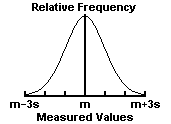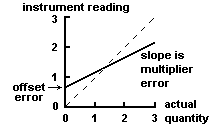# Random vs Systematic Error

## Random Errors

Random errors in experimental measurements are caused by unknown and unpredictable changes in the experiment. These changes may occur in the measuring instruments or in the environmental conditions.

Examples of causes of random errors are:

• electronic noise in the circuit of an electrical instrument,
• irregular changes in the heat loss rate from a solar collector due to changes in the wind.
Random errors often have a Gaussian normal distribution (see Fig. 2). In such cases statistical methods may be used to analyze the data. The mean m of a number of measurements of the same quantity is the best estimate of that quantity, and the standard deviation s of the measurements shows the accuracy of the estimate. The standard error of the estimate m is s/sqrt(n), where n is the number of measurements.Fig. 2. The Gaussian normal distribution. m = mean of measurements. s = standard deviation of measurements. 68% of the measurements lie in the interval m - s < x < m + s; 95% lie within m - 2s < x < m + 2s; and 99.7% lie within m - 3s < x < m + 3s.

The precision of a measurement is how close a number of measurements of the same quantity agree with each other. The precision is limited by the random errors. It may usually be determined by repeating the measurements.

## Systematic Errors

Systematic errors in experimental observations usually come from the measuring instruments. They may occur because:
• there is something wrong with the instrument or its data handling system, or
• because the instrument is wrongly used by the experimenter.
Two types of systematic error can occur with instruments having a linear response:
1. Offset or zero setting error in which the instrument does not read zero when the quantity to be measured is zero.
2. Multiplier or scale factor error in which the instrument consistently reads changes in the quantity to be measured greater or less than the actual changes.
These errors are shown in Fig. 1. Systematic errors also occur with non-linear instruments when the calibration of the instrument is not known correctly.Fig. 1. Systematic errors in a linear instrument (full line).
Broken line shows response of an ideal instrument without error.

Examples of systematic errors caused by the wrong use of instruments are:

• errors in measurements of temperature due to poor thermal contact between the thermometer and the substance whose temperature is to be found,